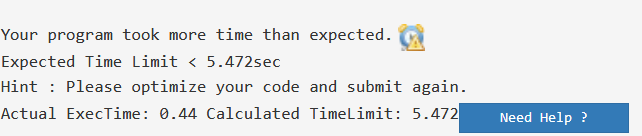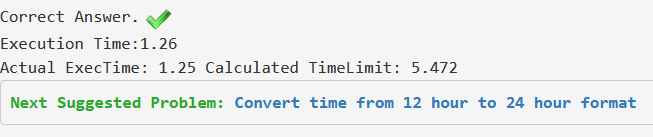# How to get rid of Java TLE problem

It happens many times that you have written correct Java code with as much optimization as needed according to the constraints. But, you get TLE 😢.
This happens due to the time taken by Java to take input and write output using Scanner class which is slow as compared to BufferedReader and StringBuffer class. Read in detail about Scanner Class here.

Have a look at some tips to get rid of this TLE issue (when your logic is correct obviously)?

Tip 1 : Avoid using Scanner Class and try to use BufferedReader class.
Tip 2 : Try to use StringBuffer class in case you have to print large number of data.

Let’s take a problem from GeeksforGeeks practice and solve the TLE issue:
Problem : Segragate an Array of 0s, 1s and 2s
In short, problem is, given an array of 0s, 1s and 2s. We have to segregate all the 0s in starting of array, all the 1s in mid of the array, and all the 2s in last of the array.
Examples:

```Input : 1 1 2 0 0 2 1
Output : 0 0 1 1 1 2 2
```

Approach : Segregate array of 0s, 1s and 2s

Below is the implementation of above Approach :

 `// Program to segragate the ` `// array of 0s, 1s and 2s ` `import` `java.util.*; ` `import` `java.lang.*; ` `import` `java.io.*; ` `class` `GFG { ` `    ``public` `static` `void` `main(String[] args) ` `    ``{ ` `        ``// Using Scanner class to take input ` `        ``Scanner sc = ``new` `Scanner(System.in); ` ` `  `        ``// Number of testcase input ` `        ``int` `t = sc.nextInt(); ` ` `  `        ``// Iterating through all the testcases ` `        ``while` `(t-- > ``0``) { ` ` `  `            ``// Input n, i.e. size of array ` `            ``int` `n = sc.nextInt(); ` ` `  `            ``int` `arr[] = ``new` `int``[n]; ` ` `  `            ``// Taking input of array elements ` `            ``for` `(``int` `i = ``0``; i < n; i++) ` `                ``arr[i] = sc.nextInt(); ` ` `  `            ``// Calling function to segragate ` `            ``// input array ` `            ``segragateArr(arr, n); ` ` `  `            ``// printing the modified array ` `            ``for` `(``int` `i = ``0``; i < n; i++) { ` `                ``System.out.print(arr[i] + ``" "``); ` `            ``} ` ` `  `            ``System.out.println(); ` `        ``} ` `        ``sc.close(); ` `    ``} ` ` `  `    ``// Function to segragate 0s, 1s and 2s ` `    ``public` `static` `void` `segragateArr(``int` `arr[], ``int` `n) ` `    ``{ ` `        ``/* ` `        ``low : to keep left index ` `        ``high : to keep right index ` `        ``mid : to get middle element ` `        ``*/` `        ``int` `low = ``0``, high = n - ``1``, mid = ``0``; ` ` `  `        ``// Iterating through the array and ` `        ``// segregating elements ` `        ``while` `(mid <= high) { ` ` `  `            ``// If element at mid is 0 ` `            ``// move it to left ` `            ``if` `(arr[mid] == ``0``) { ` `                ``int` `temp = arr[low]; ` `                ``arr[low] = arr[mid]; ` `                ``arr[mid] = temp; ` `                ``low++; ` `                ``mid++; ` `            ``} ` ` `  `            ``// If element at mid is 1 ` `            ``// nothing to do ` `            ``else` `if` `(arr[mid] == ``1``) { ` `                ``mid++; ` `            ``} ` ` `  `            ``// If element at mid is 2 ` `            ``// move it to last ` `            ``else` `{ ` `                ``int` `temp = arr[mid]; ` `                ``arr[mid] = arr[high]; ` `                ``arr[high] = temp; ` `                ``high--; ` `            ``} ` `        ``} ` `    ``} ` `} `

According to our expectations, it should pass all the testcases and get accepted on GeeksforGeeks practice. But, when we submit this code on GeeksforGeeks IDE, it shows TLE.This signifies that we have exceeded the time limit as expected. Not an issue, let’s use the tips given above.

1. Use BufferedReader to take input.
2. Use StringBuffer to save and print output.

Approach : Segregate array of 0s, 1s and 2s

Below is the implementation of Java code for segregating 0s, 1s and 2s

 `// Java program to segragate ` `// array of 0s, 1s and 2s ` `import` `java.io.*; ` `import` `java.util.*; ` ` `  `class` `GFG { ` `    ``// Driver Code ` `    ``public` `static` `void` `main(String[] args) ``throws` `IOException ` `    ``{ ` ` `  `        ``// Using BufferedReader class to take input ` `        ``BufferedReader br = ``new` `BufferedReader(``new` `InputStreamReader(System.in)); ` ` `  `        ``// taking input of number of testcase ` `        ``int` `t = Integer.parseInt(br.readLine()); ` ` `  `        ``while` `(t-- > ``0``) { ` `            ``// n : size of array ` `            ``int` `n = Integer.parseInt(br.readLine()); ` ` `  `            ``// Declaring array ` `            ``int` `arr[] = ``new` `int``[n]; ` ` `  `            ``// to read multiple integers line ` `            ``String line = br.readLine(); ` `            ``String[] strs = line.trim().split(``"\\s+"``); ` ` `  `            ``// array elements input ` `            ``for` `(``int` `i = ``0``; i < n; i++) ` `                ``arr[i] = Integer.parseInt(strs[i]); ` ` `  `            ``// Calling Functions to segregate Array elements ` `            ``segragateArr(arr, n); ` ` `  `            ``// Using string buffer to append each output in a string ` `            ``StringBuffer sb = ``new` `StringBuffer(); ` `            ``for` `(``int` `i = ``0``; i < n; i++) ` `                ``sb.append(arr[i] + ``" "``); ` ` `  `            ``// finally printing the string ` `            ``System.out.println(sb); ` `        ``} ` `        ``br.close(); ` `    ``} ` ` `  `    ``// Function to segragate 0s, 1s and 2s ` `    ``public` `static` `void` `segragateArr(``int` `arr[], ``int` `n) ` `    ``{ ` `        ``/* ` `        ``low : to keep left index ` `        ``high : to keep right index ` `        ``mid : to get middle element ` `        ``*/` `        ``int` `low = ``0``, high = n - ``1``, mid = ``0``; ` ` `  `        ``// Iterating through the array and ` `        ``// segregating elements ` `        ``while` `(mid <= high) { ` ` `  `            ``// If element at mid is 0 ` `            ``// move it to left ` `            ``if` `(arr[mid] == ``0``) { ` `                ``int` `temp = arr[low]; ` `                ``arr[low] = arr[mid]; ` `                ``arr[mid] = temp; ` `                ``low++; ` `                ``mid++; ` `            ``} ` ` `  `            ``// If element at mid is 1 ` `            ``// nothing to do ` `            ``else` `if` `(arr[mid] == ``1``) { ` `                ``mid++; ` `            ``} ` ` `  `            ``// If element at mid is 2 ` `            ``// move it to last ` `            ``else` `{ ` `                ``int` `temp = arr[mid]; ` `                ``arr[mid] = arr[high]; ` `                ``arr[high] = temp; ` `                ``high--; ` `            ``} ` `        ``} ` `    ``} ` `} `Great! You have leveled up.
Java TLE issue? Seems pretty much simple :). You may try it now.My Personal Notes arrow_drop_upCheck out this Author's contributed articles.

If you like GeeksforGeeks and would like to contribute, you can also write an article using contribute.geeksforgeeks.org or mail your article to contribute@geeksforgeeks.org. See your article appearing on the GeeksforGeeks main page and help other Geeks.

Please Improve this article if you find anything incorrect by clicking on the "Improve Article" button below.

Improved By : patrick.1729

Article Tags :
Practice Tags :

19

Please write to us at contribute@geeksforgeeks.org to report any issue with the above content.